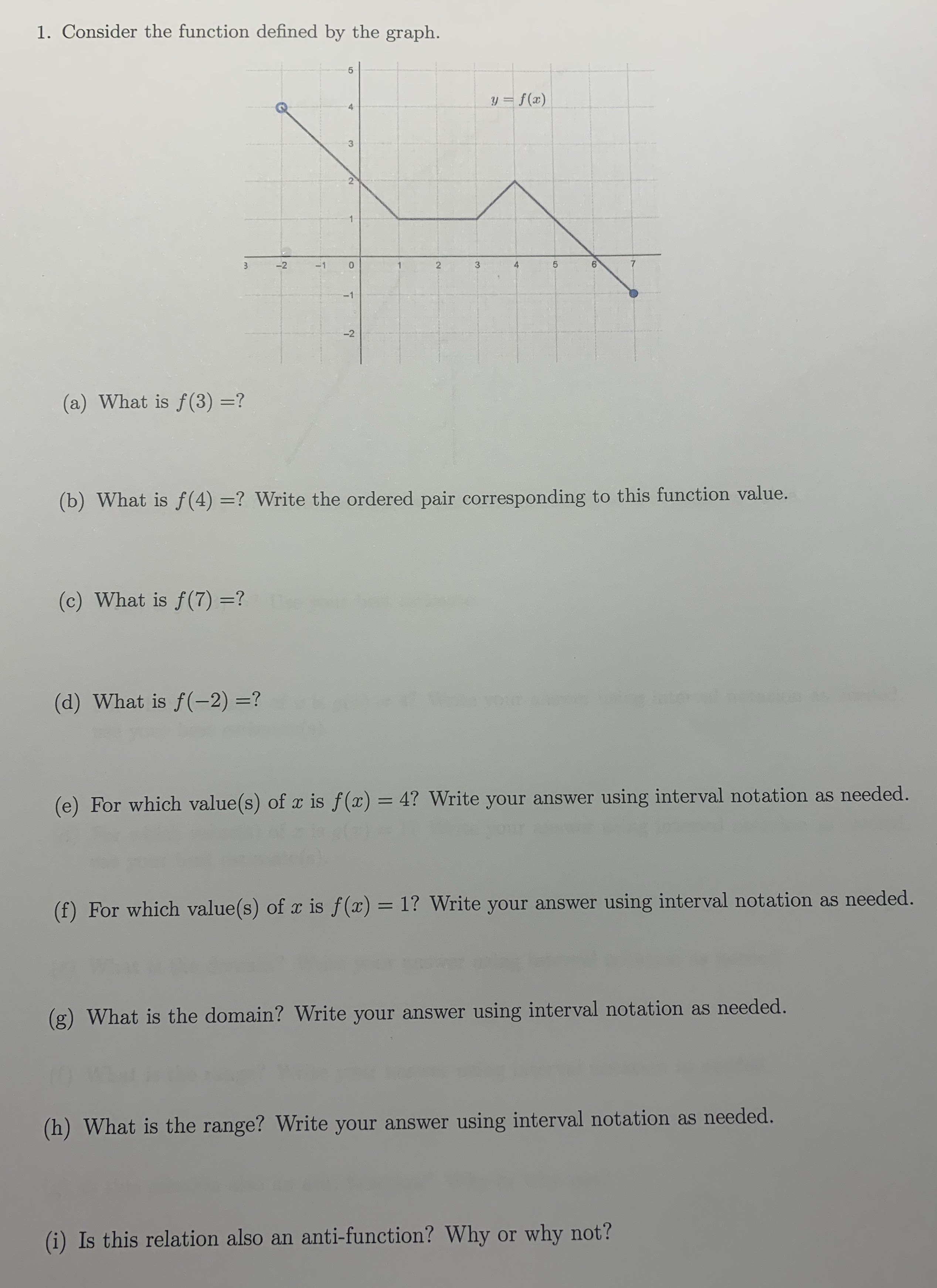### ¿Todavía tienes preguntas de matemáticas?

Pregunte a nuestros tutores expertos
Algebra
Pregunta1. Consider the function defined by the graph. (a) What is $$f ( 3 ) =$$ ? (b) What is $$f ( 4 ) =$$ ? Write the ordered pair corresponding to this function value. (c) What is $$f ( 7 ) =$$ ? (d) What is $$f ( - 2 ) =$$ ? (e) For which value(s) of $$x$$ is $$f ( x ) = 4$$ ? Write your answer using interval notation as needed. (f) For which value(s) of $$x$$ is $$f ( x ) = 1$$ ? Write your answer using interval notation as needed. (g) What is the domain? Write your answer using interval notation as needed. (h) What is the range? Write your answer using interval notation as needed. (i) Is this relation also an anti-function? Why or why not?

f(3)=1

f(4)=2

f(7)=-1

f(-2)=4

f(x)=4, x=-2

f(x)=1, x=1, 2, 3, 5

Solución
View full explanation on CameraMath App.